# SAT Math Multiple Choice Question 493: Answer and Explanation

### Test Information

Question: 493

13.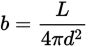The brightness of a celestial body, like a star, decreases as you move away from it. In contrast, the luminosity of a celestial body is a constant number that represents its intrinsic brightness. The inverse square law, shown above, is used to find the brightness, b, of a celestial body when you know its luminosity, L, and the distance, d, in meters to the body. Which equation shows the distance to a celestial body, given its brightness and luminosity?

• A.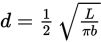• B.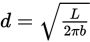• C.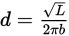• D.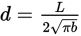Explanation:

A

Difficulty: Medium

Category: Passport to Advanced Math / Exponents

Strategic Advice: Don't spend too much time reading the scientific explanation of the equation. Focus on the question at the very end—it's just asking you to solve the equation for d.

Getting to the Answer: First, cross-multiply to get rid of the denominator. Then, divide both sides of the equation by 4πb to isolate d 2. Finally, take the square root of both sides to find d.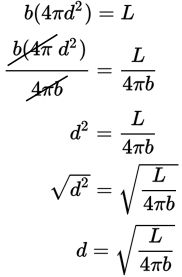Unfortunately, this is not one of the answer choices, so you'll need to simplify further. You can take the square root of 4 (it's 2), but be careful—it's in the denominator of the fraction, so it comes out of the square root as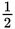.

The simplified equation is.